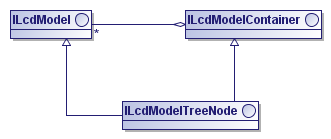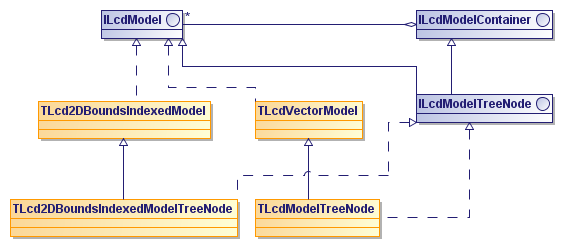In a similar way as you can group layers, which is discussed in Grouping layers, you can also group models. You may find this useful to group models containing related data sets. LuciadLightspeed provides the following options to group a set of models in a hierarchical model structure:

• Create a model list with submodels using the class `TLcdModelList`. A model list is a composite model. As such applications can handle a model list as a single model regardless of its submodels. Creating a model list provides more details on creating and using a model list.

• Create a model node with child models using the interface `ILcdModelTreeNode`. A model node is a model itself. Applications consider the model node and its child models as separate models. A `TLcdZipModelListDecoder` for example, creates a model node which contains models for each data set included in a given zip file. Creating a model node provides more details on creating and using a model node.

## Creating a model list

The class `TLcdModelList` allows you to create an `ILcdModel` that is composed of an ordered list of other `ILcdModel` objects, based on the Composite design pattern. Each added model, or submodel, can be a `TLcdModelList` itself. Using a `TLcdModelList` instead of adding all elements (domain objects) of the submodels to a flat model, allows fast reordering of the submodels once the model list has been created. Additionally, each submodel can have its own model descriptor, next to the model descriptor of the model list. Note however that when you use a `TLcdEditableModelListDescriptor` for the model list, the model descriptor is automatically updated whenever a submodel is added or removed from the model list. Note also that since a `TLcdModelList` is an `ILcdModel`, all of the submodels should have the same model reference.

To enumerate the elements of the models in a model list you can use the method `elements()`. This method iterates the submodels in the list one by one. Note that a `TLcdModelList` itself is not an editable model; you cannot add or remove domain objects to it.

 If all submodels implement `ILcd2DBoundsIndexedModel`, it is best to use the subclass `TLcd2DBoundsIndexedModelList` so you can perform spatial queries on the model list.

Another option for grouping models is creating an `ILcdModelTreeNode` as described in Creating a model node. The main difference between a model list and a model node is that a model list is a composite model and a model node is a model itself that can have child models.

## Creating a model node

Similarly to creating a layer node, as described in Grouping layers, you can group models in a model (tree) node by using the interface `ILcdModelTreeNode`. As Figure 1, “Class diagram of `ILcdModelTreeNode`” shows, an `ILcdModelTreeNode` is an `ILcdModel` that also implements `ILcdModelContainer` which allows you to add child models to the model node.Figure 1. Class diagram of `ILcdModelTreeNode`

Since the model node is a model itself, all methods inherited from `ILcdModel` only apply to the model node and not to its child models. For example, calling `addElement(Object, int)` results in the addition of the given element (domain object) to the model node, and not to its child models. On the other hand, the methods inherited from `ILcdModelContainer` only apply on the child models. Calling `modelCount()` on the model node, for example, returns the number of child models of the model node, not including the node itself.

The difference with a `TLcdModelList` is that a model list is a composite model and a model node is a model itself that can have child models. As a consequence, the methods inherited from `ILcdModel` behave differently on the model node than on the model list. For example, calling `ILcdModel.elements()` on a model node only returns the elements of the model node and not of its child models. Calling the method on a model list returns the elements of all the submodels of the model list. Also, each of the child models of a model node can have a different model reference, contrary to the submodels of a model list.

 A model node can contain domain objects itself but you can also use it as a mere container for other models. A model node without domain objects is empty and should thus return true for `isEmpty()`.

In addition to the methods inherited from `ILcdModelContainer`, to add/remove child models and retrieve a specific model, the `ILcdModelTreeNode` has methods to add a hierarchical listener of the type `ILcdModelListener` and `ILcdModelContainerListener`. These listeners receive events for all changes to the models and model nodes contained in the hierarchical model structure. For more information on listeners, refer to Notifying objects of changes with listeners.

As shown in Figure 2, “Main implementations of `ILcdModelTreeNode`”, `ILcdModelTreeNode` has two main implementations: `TLcdModelTreeNode` and `TLcd2DBoundsIndexedModelTreeNode`. As long as the model node itself contains no domain objects but only child models, it is best to use the `TLcdModelTreeNode`. For model nodes that contain domain objects itself, it is more efficient to use an `ILcd2DBoundsIndexedModel` such as the `TLcd2DBoundsIndexedModelTreeNode`.Figure 2. Main implementations of `ILcdModelTreeNode`